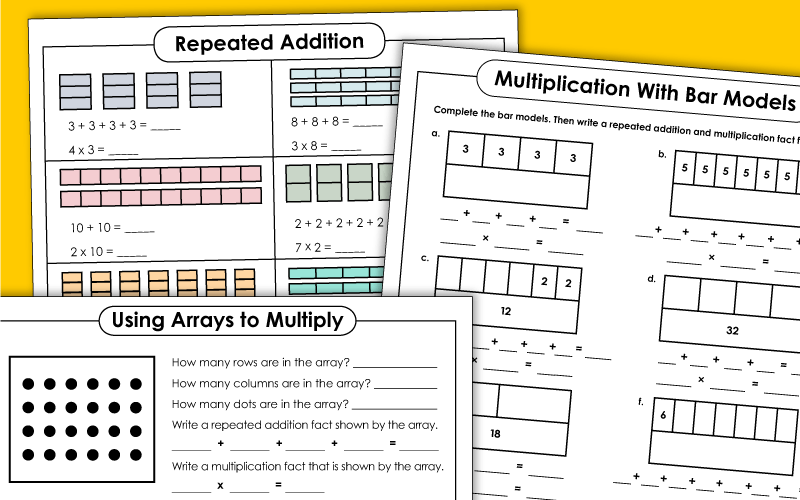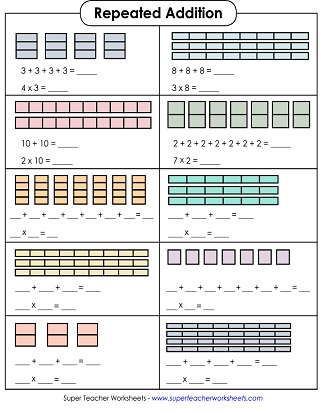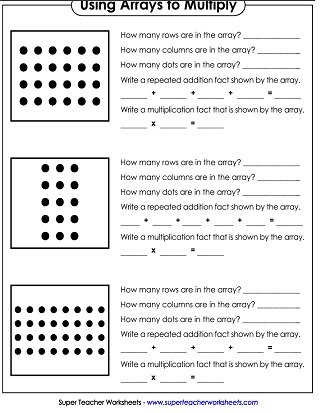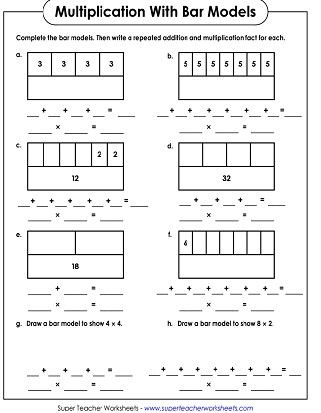This page has printable worksheets and activities for reviewing repeated addition. This skill is typically taught as a prerequisite to basic multiplication.Use repeated addition to find the number of fruits shown. Write each as a multiplication fact and as a repeated addition equation.
The models show repeated addition equations as rectangular boxes. Write the equation for each.
Write a multiplication fact and repeated addition number sentence for each illustration.

Tell how many rows and columns are in each array. hen write a repeated addition problem, as well as a multiplication fact.
Write a repeated addition fact and multiplication fact for each array shown.

## Repeated Addition w/ Bar Models

Analyze each bar model. Write numbers in the empty blocks. Then write a repeated addition number sentences and a multiplication fact for each.
This is a slightly easier version of the bar model worksheet above.
Basic Multiplication

Teaching basic multiplication with our PDF worksheets, task cards, flash cards, class games, and cut-and-glue activities.

STW has thousands of printable worksheets for teaching basic addition skills.

## Sample Worksheet ImagesMy Account
Site Information